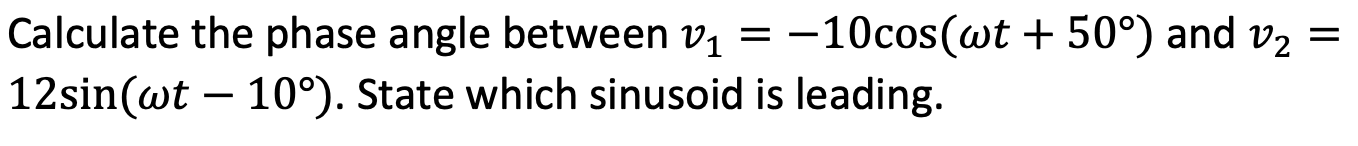# Question Solved2 AnswersCalculate the phase angle between V1 = -10cos(wt + 50°) and v2 = 12sin(wt – 10°). State which sinusoid is leading.9WOVER The Asker · Electrical EngineeringTranscribed Image Text: Calculate the phase angle between V1 = -10cos(wt + 50°) and v2 = 12sin(wt – 10°). State which sinusoid is leading.
More
Transcribed Image Text: Calculate the phase angle between V1 = -10cos(wt + 50°) and v2 = 12sin(wt – 10°). State which sinusoid is leading.